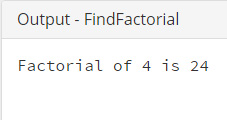# Java Program to Find Factorial

This Java program is used to find the factorial.

Factorial of any number is !n.
For example, factorial of 4 is 4*3*2*1.

Example:
``````public class FindFactorial {

public static void main(String[] args) {

int number = 4;
int factorial = number;

for (int i = (number - 1); i & gt; 1; i--) {
factorial = factorial * i;
}

System.out.println("Factorial of " + number + " is " + factorial);
}
}``````
Program Output:Explanation:

Here is a detailed explanation of what is happening within this code snippet -

This program will find out the factorial for a number, a classic is declared named FactorialNumber is declared with the keyword public. Public designates that the class can be accessible from anywhere within the program. Within this class, the main() method is invoked. The main() method is having two variables of String class. These are:

• int number = 4;
• int factorial = number;

Here, the two variables are storing the string value 2 integer type variales.

Now, a loop has to be implemented (here for loop) and within thisloop, the county variable 'i' is initialized as number-1, and the loop will continue till (i>1).

Then the statement factorial =factorial * i; is given which calculates the factorial taking one value of 'i' at a time within loop and storing them back in the 'factorial' variable. This loop will start from one value which is number minus 1 and based on the condition, the loop will decrement and comes to 1.

Finally, the 'factorial' variable has been printed using the System.out.println().